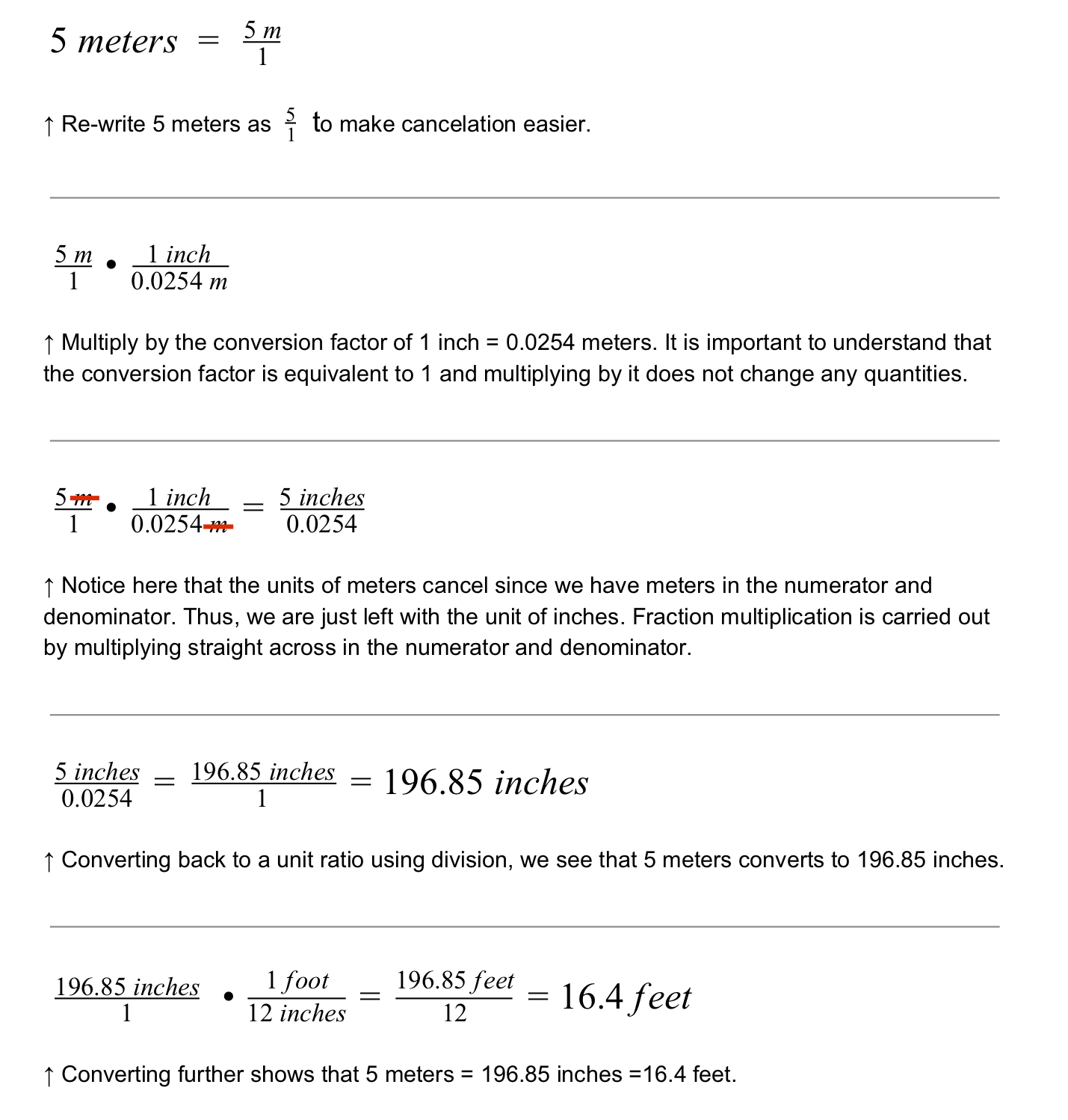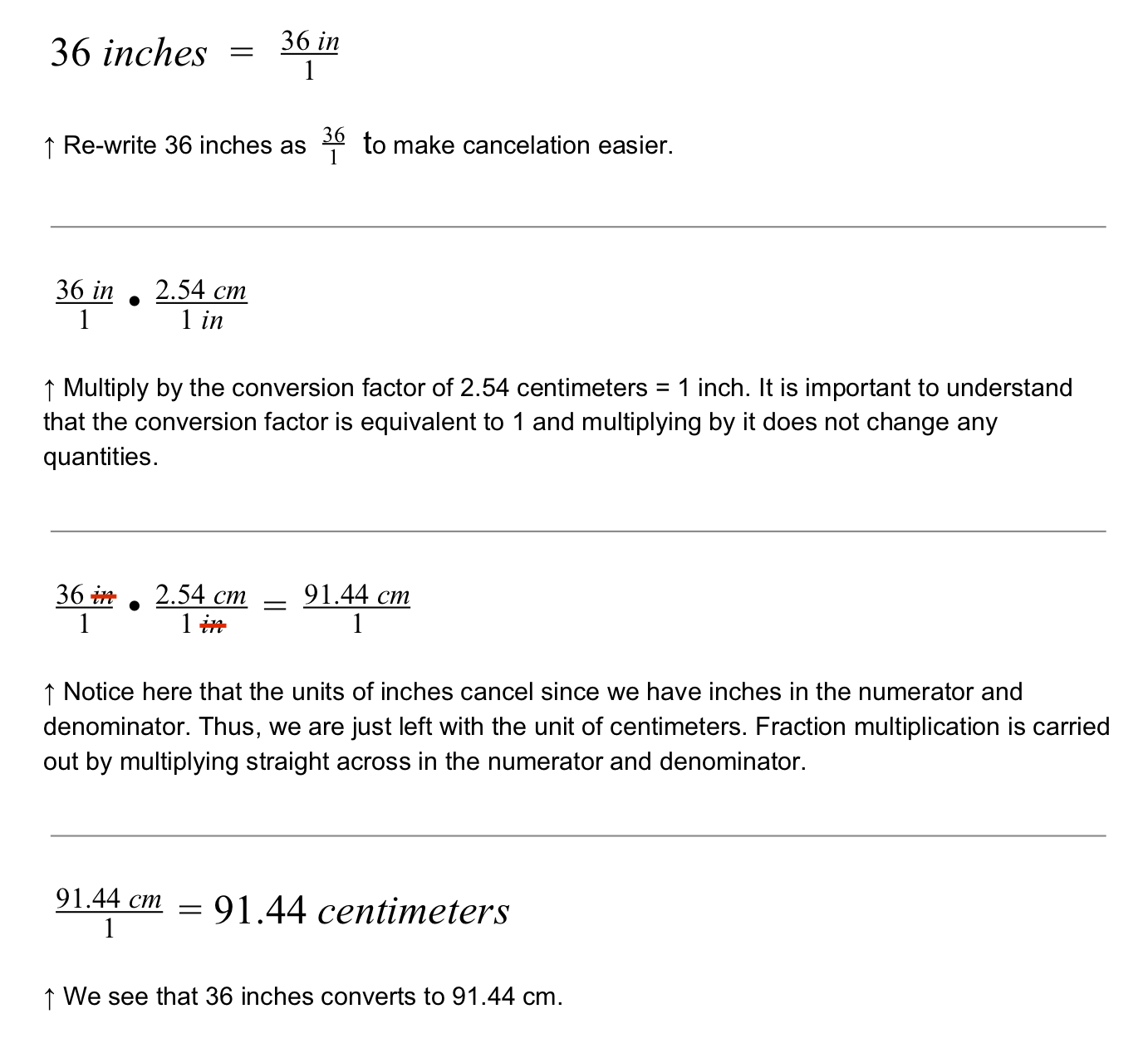STEMLabs V5
Robo Rally Teacher

Teacher PortalTeacher Toolbox - The Purpose of This Page

Later in this Play section the students will be asked to calculate and draw a scaled version of a Race Course that they will design. In order to scale-down the dimensions of the real-life Race Course, they must be able to convert units. More specifically, they will be measuring the actual dimensions of the area they can use for the course in larger units such as meters or feet. They must then be able to scale these units down into smaller units such as millimeters, centimeters or inches so that the drawing in their engineering notebook is proportional to the actual sized course. The following reading and questions provides an overview of conversion factors and how they can be used for unit conversions.

Read through the following conversions on this page as a whole class. For the Motivate Discussion section, ask the students to attempt the questions first on their own in their engineering notebooks. Once all of the students have had time to attempt the questions, engage the students in a whole class discussion to review their solutions.

Vocabulary

The following mathematical vocabulary will be used throughout this STEM Lab:

• Proportion: When two ratios are equal.

• Ratio: A mathematical comparison of two values.

• Unit Ratio: A ratio with a denominator of 1.

• Conversion Factor: An expression for the equal exchange between units.

• Unit Conversion: The process of converting a measurement in one set of units to the same measurement in another set of units.

• Scale: The relationship or ratio between a set distance on a map, model or drawing and the corresponding measurement on the actual object.

• Scale Drawing: The drawing of an object that is proportional.

### Changing Units Without Changing Measurements

Sometimes, different designers may use different sets of units. For example, much of the world uses metric units like meters and centimeters, but a few places in the world uses imperial units like feet and inches. If we have different ways of measuring things, we could easily get the wrong measurements!

When converting between measurements, it is helpful to use conversion factors. Here are some examples:

• 1 meter = 100 centimeters = 1000 millimeters

• 1 inch = 2.54 centimeters = 25.4 millimeters = 0.0254 meters

• 1 yard = 3 feet = 36 inches = 914.4 millimeters

• 1 kilogram = 1000 grams

• 1 liter = .001 cubic meters

How many inches is 5 meters? Use the conversion factor 1 inch = 0.0254 meters.How many centimeters is 36 inches? Use the conversion factor 1 in = 2.54 cm.Motivate Discussion

Give your students an opportunity to solve other conversion examples and to explain their reasoning for how they calculated those conversions. Not all of your students will readily understand the calculations above as written. Reasoning through them orally might be helpful.
Q: A piece of your robot weighs 2500 grams. How many kilograms does it weigh? Explain.
A: If 1 kilogram is equal to 1000 grams, then I need to know how many 1000s (the number of grams in a kilogram) are in 2500 (the total weight of the piece). I divide 2500 grams by 1000 grams (1 kg) and find that my piece weights 2.5 kilograms.

Q: We know from this page that 1 inch equals 2.54 centimeters and 1 yard equals 3 feet. About how many centimeters are in a yard? Explain.
A: If 1 yard equals 3 feet, then there are 36 inches (12 inches x 3 = 36 inches) in a yard.
Because 1 inch equals 2.54 centimeters, I then multiply 36 (inches in a yard) by 2.54 (centimeters in an inch) and find 91.44 centimeters are in a yard.

Q: As a follow-up question, about how many inches are in a meter? Explain.
A: If 1 meter equals 100 centimeters and 1 centimeter is equal to 2.54 inches, then I need to know how many times 2.54 goes into 100. Divide 100 (the number of centimeters in a meter) by 2.54 (the number of centimeters in an inch) and find that there are about 39.37 inches in a meter.